# Extraction of a Root

The following article is from The Great Soviet Encyclopedia (1979). It might be outdated or ideologically biased.

## Extraction of a Root

the inverse algebraic operation to raising to a power. To extract an nth root of a number a means to find a number (or numbers) x that, when raised to the nth power, will yield the given number a (xn = a). The number x (written as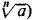) is called the root, n is called the index of the root, and a is called the radicand. The symbol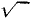is an altered version of the letter r (from the Latin radix, “root”). If we restrict ourselves to real numbers, then for example,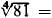= ±3, since (± 3)4 = 81; but if we allow complex numbers, then there are two more roots,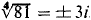. We speak of a square root (designated as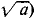) rather than a second root and of a cube root rather than a third root. The problem of extracting an nth root of a number a is equivalent to solving the binomial equation xna = 0. Over the complex numbers, this equation has n solutions, and consequently there exist n roots of the number a. If a is a positive real number, then one of the roots will also be real and positive; in that case, the problem of extracting the root often means finding the positive real root. Roots of rational numbers are not always rational, and therefore the problem of finding their approximate values arises. Logarithmic tables or special tables of roots are used in calculating roots.

### REFERENCES

Bradis, V. M. Chetyrekhznachnye matematicheskie tablitsy, 41st ed. Moscow, 1970.
Barlou, P. Tablitsy kvadratov, kubov, kvadratnykh kornei, kubicheskikh kornei i obratnykh velichin vsekh tselykh chisel do 12500. Moscow, 1965.
The Great Soviet Encyclopedia, 3rd Edition (1970-1979). © 2010 The Gale Group, Inc. All rights reserved.
Site: Follow: Share:
Open / Close